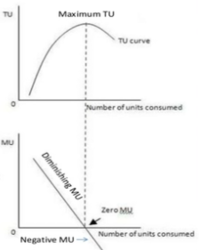# Concept of Total Utility and Marginal UtilityTotal Utility:
It is the total  satisfaction derived from consumption of given quantity of a commodity at a given time. In other words, It is the sum total of marginal utility.Marginal Utility:
It is the change in total utility resulting from the consumption of an additional unit of the commodity. In other words, It is the utility derived from each additional unit.Relationship between Total Utility (TU) and Marginal Utility(MU)
The relationship between total utility and marginal utility can be understood with the help of table given below:

 Units of Orange Total Utility Marginal Utility Description 1234 8141820 8642 TU is increasing and MU is positive 5 20 0 TU is maximum and stable; and MU is zero. 6 18 -2 TU is decreasing and MU is positive.

In the above table, total utility increases at a decreasing rate up to the 4th unit of orange consumed and hence marginal utility decreases. As the consumer consumes the 5th unit of orange, total utility becomes maximum and marginal utility is equal to zero. As consumption of orange increases to the 6th unit, total utility begins to decline and marginal utility begins to decline and marginal utility becomes negative, i.e. -2 utils. It can be graphically explained with the help of the figure.In the  figure, units of consumption and utility are measured along the X-axis and Y-axis respectively. For each combination of units of consumption and marginal utility, we plot a point on the graph. Joining these points, we obtain a MU curve indicating marginal utility curve. It slopes downward from left to right which indicating that MU declines as a consumer consume more and more units of the same commodity. Similarly, for each combination of units of consumption and total utility we plot a point on the graph. Joining these points, we obtain a TU curve indicating total utility curve. Initially, it increases at a diminishing rate then reaches its maximum and then starts declining.
The relationship between total utility (TU) and marginal utility(MU) can be summarized as follows:
1. TU is the sum of total of MU whereas MU is the change in TU.
2. TU generally remains positive while MU may be positive, zero or even negative.
3. When MU is positive, TU increases.
4. When MU is zero, TU is maximum.
5. When MU is negative, TU starts diminishing.